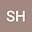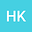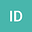An objective matched-filter technique
•••Hironori Kawakata
Ritsumeikan University, Ritsumeikan University
Author Profile## Abstract

We propose an objective threshold determination method for detecting outliers from the empirical distribution of cross-correlation coefficients among seismic waveforms. This method is aimed at detecting seismic signals from continuous waveform records. In our framework, detectability is automatically determined from Akaike’s Information Criterion (AIC). We applied the method of seismic signal detection to continuous records collected over 2 years. The results show that the maximum value of network cross-correlation coefficients sampled from each constant interval can be approximated by the theory of extreme value statistics, which provides a parametric probability density function of maxima. Using the function, outliers can be considered with a reasonable criterion.